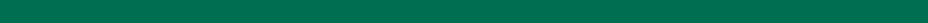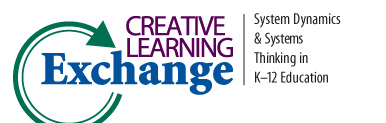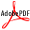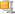>
CLE Curriculum Search
Search results for: Diana M. Fisher
28 records found. Currently displaying page 2 of 3 [<< Prev] 1 2 3 [Next >>]
 Lessons in Mathematics Section 5: Oscillatory Behavior Author(s): Diana M. Fisher Subject: Math This book provides a set of tools that enables educators to teach mathematics using the framework of System Dynamics. Section 5 covers sinusoidal functions, including simple harmonic motion. Distance versus time lessons using a motion detector are also inPDFMore about the book at: http://www.iseesystems.com/store/college_university/MathBook.aspx Lessons in Mathematics Section 6: Convergent and Logistic Behavior Author(s): Diana M. Fisher Subject: Math This book provides a set of tools that enables educators to teach mathematics using the framework of System Dynamics. Section 6 takes students through the steps from exponential to convergent to logistic models, culminating with an application to a systemPDFMore about the book at: http://www.iseesystems.com/store/college_university/MathBook.aspx Lessons in Mathematics Section 7: Differential Equations Author(s): Diana M. Fisher Subject: Math This book provides a set of tools that enables educators to teach mathematics using the framework of System Dynamics. Section 7 builds on the skills of Sections 5 and 6 using classic problems studied with differential equations. Exponential, convergent anPDFMore about the book at: http://www.iseesystems.com/store/college_university/MathBook.aspx Lessons in Mathematics Section 8: Miscellaneous Topics Author(s): Diana M. Fisher Subject: Math This book provides a set of tools that enables educators to teach mathematics using the framework of System Dynamics. Section 8 presents a natural extension of linear and exponential growth study - arithmetic and geometric sequences. Application to an age More about the book at: http://www.iseesystems.com/store/college_university/MathBook.aspx Lessons in Mathematics Section 9: Appendix Author(s): Diana M. Fisher Subject: Math This book provides a set of tools that enables educators to teach mathematics using the framework of System Dynamics. Section 9 is a useful reference for all lessons in the book. Included are generic modeling structures, a summary of motion, distance-velo More about the book at: http://www.iseesystems.com/store/college_university/MathBook.aspx Lessons in Mathematics: A Dynamic Approach Author(s): Diana M. Fisher Subject: Math The abstractness of a traditional approach to mathematics causes many students to falter. This book focuses on making the abstractness more concrete with interesting and fun lessons. It uses STELLA software's icon-based, non-abstract language to structure More about the book at: http://www.iseesystems.com/store/college_university/MathBook.aspx Linear Models in STELLA Author(s): Diana M. Fisher, & Rogers Subject: Math From CC-STADUS. Introductory tutorial exercises using STELLA II for high school math. Involves distance, car depreciation and jumping out of an airplane.Zipped (Models & PDF) Linear Systems Using STELLA Author(s): Diana M. Fisher Subject: Math From CC-STADUS. Semi-complex STELLA tutorial. Involves train robbery, Star Trek, and camping.PDF Modeling Dynamic Systems Section 10 Author(s): Diana Fisher Subject: System Dynamics There are several characteristics of complex systems that can be discussed with students during these lessons. The fictitious city seems to face a dilemma; the tanning industry provides needed jobs in the present, but water pollution can cause serious detPDFLink to the simulation: http://www.iseesystems.com Modeling Dynamic Systems Section 6 Author(s): Diana Fisher Subject: System Dynamics In the classroom example provided in this section, the author lists many "potential systems problems" that are related to the issue of overpopulation. As an extension exercise, students can be asked to identify ways in which people have attempted to solvePDFLink to the simulation: http://www.iseesystems.com
Sorted Ascending by Title Hide Descriptions